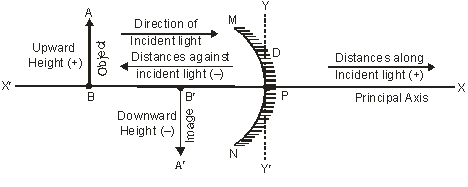# 10th Physics | Light | Sign Convention for Reflection by Spherical Mirrors and Mirror Formula

## Sign Convention for Reflection by Spherical Mirrors

The new Cartesian sign convention is used for measuring the various distances in the ray-diagrams of spherical mirrors.

According to the new Cartesian sign convention (figure 1) :

(i) The pole ‘P’ of the mirror is taken as the origin and the principal axis of the mirror is taken as the x-axis of the coordinate system.

(ii) The object is always placed to the left of the mirror i.e. the light (incident rays) from the object falls on the mirror from the left hand side.

(iii) All the distances parallel to the principal axis of the spherical mirrors are measured from the pole ‘P’ of the mirror.

(iv) All the distances measured to the right of the origin (along +ve x-axis) are taken as positive.

(v) All the distances measured to the left of the origin (along –ve x-axis) are taken as negative.

(vi) The distances (heights) measured upwards (i.e. above the x-axis) and perpendicular to the principal axis of the mirror are taken as positive

(vii) the distances (heights) measured downwards (i.e. below the x-axis) and perpendicular to the principal axis of the mirror are taken as negative.

The following fig. 32 illustrates all the points of the new cartesian sign convention stated above.Figure 1

## Mirror Formula

Let us first know about the terms used in the mirror formula of spherical mirrors.

(i) Object distance (u) : The distance of the object from the pole ‘P’ of the spherical mirror is called the object distance. It is denoted by the letter ‘u’

(ii) Image Distance (v) : The distance of the image from the pole ‘P’ of the spherical mirror is called the image distance. It is denoted by the letter ‘v’.

(iii) Focal length (f) : The distance of the principal focus (F) from the pole (P) of the spherical mirror is called the focal length. It is denoted by the letter ‘f ‘.

The relationship between the image distance (v), object distance (u) and focal length (f ) of a spherical mirror is known as the mirror formula. The Mirror formula can be written as :$\displaystyle \frac{1}{\text{Image }\ \text{distance}}+\frac{1}{\text{object}\ \text{distance}}=\frac{1}{\text{focal}\ \text{length}}$

symbolically$\displaystyle \frac{1}{v}+\frac{1}{u}=\frac{1}{f}$

where the symbols have their usual meaning.

This site uses Akismet to reduce spam. Learn how your comment data is processed.